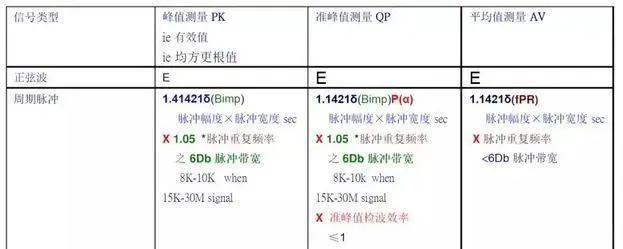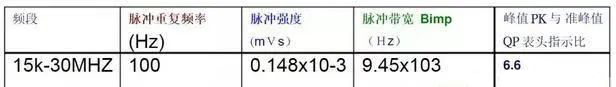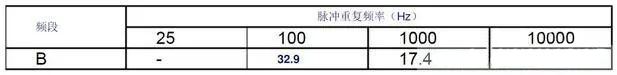Home > News > Industry News

# EMI peak, quasi-peak, average value difference analysis

## Difference analysis of EMI peak, quasi-peak and average

EMI Receiver can perform quasi-peak measurement, peak measurement and average measurement. In the EMI peak, quasi-peak and average difference analysis, when the input signal is a sine wave, the readings obtained are the same regardless of the way of measurement, equal to the effective value of the sine wave, and the accuracy should be better than ± 2dB. However, if the input is a periodic pulse signal, the readings obtained by the three measurement methods are different, and the results are shown in the table.

Comparison of measurement results of peak, quasi-peak and average values in EMI peak, quasi-peak and average value difference analysis:In the table

E - effective value of sine wave;

δ—— Pulse intensity, equal to pulse amplitude × Pulse width sec, unit: mVs

Bimp -- pulse width; Bimp="1". 05B6 (B6 represents pulse bandwidth of 6Db bandwidth, at 15k-30MHZ, 8khz<B6<10khz)

PP( α)—— Quasi-peak detection efficiency is related to the charging and discharging time constant, pulse repetition rate and bandwidth of the detector, P（ α)≤ 1。

FPR -- pulse repetition frequency;

It can be seen from the table that the peak measurement result ≥ the quasi-peak measurement result in the EMI peak, quasi-peak and average difference analysis. The following table lists the ratio of peak to quasi-peak meter head indication of input standard pulse under standard broadband. Excellent pulse characteristics during peak measurementThe following table lists the ratio of the quasi-peak QP and the average AV indicator with the same bandwidth, and the ratio of the quasi-peak and average indicator readings under the same bandwidthIt can be seen from the table that in the differential analysis of EMI peak value, quasi-peak value and average value, quasi-peak value ≥ average value. For regular periodic pulse, the conversion between peak value, quasi-peak value and average value can be carried out according to Table 7. However, the general disturbance is random and it is difficult to convert each other. Therefore, some standards also specify the quasi-peak limit and average limit of emission measurement.

keywords： EMI
Products Recommended
Latest Information